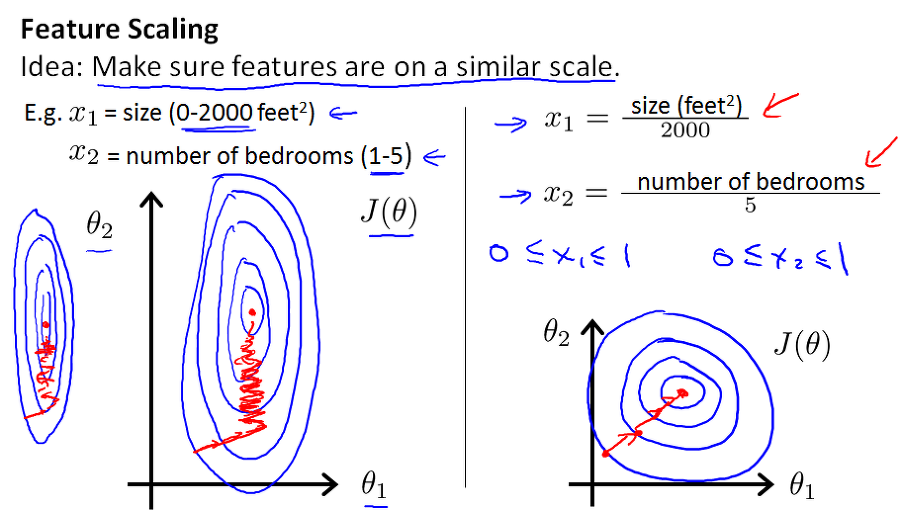# Batch Normalization

Categories:

Updated:

Batch normalization is basically normalizing units of each layer; that is controlling mean and variance of each feature vector of a layer. It can be applied either before or after activation.

Let $Z(\in R^{m \times n})$ be a output of linear transformation of a particular layer in neural networks. Also, let’s say square or square-root calculations are done element-wise.

1. $\mu(\in R^n) = \frac{1}{m}\sum_iZ^{(i)}$

2. $\sigma^2(\in R^n)=\frac{1}{m}\sum_i(Z^{(i)}-\mu)^{2}$

3. $Z_{norm}(\in R^{m\times n})=\frac{Z-\mu}{\sqrt{\sigma^2+\epsilon}}$

4. $\tilde{Z}(\in R^{m\times n}) = Z_{norm}\gamma+\beta$
Note that $\gamma, \beta \in R^n$.

5. use $\tilde{Z}$ as an input of activation layer instead of $Z$.

Note that we do not need parameter $b$ in the layer that we’ve applied batch normalization. Remember that applying bias added same value to elements of each feature vector. Thus normalizing each feature vector will offset bias.

### Why use $\gamma, \beta$?

$\gamma$ controls variance and $\beta$ controls mean.

If $\gamma = \sqrt{\sigma^2+\epsilon}$ and $\beta = \mu$, $\tilde{Z}$ equals $Z$. If $\gamma$ gets bigger, variance gets smaller. If $\beta$ gets bigger, mean also gets bigger.

$\gamma$ and $\beta$ are trainable parameters like $W$ or $b$. We can use back-prop to optimize $\gamma$ and $\beta$.

# Why Batch Normalization?

Adding batch normalization layer before or after activation layer helps neural network perform better.

## 1. Speeds Up Gradient Descent

Weights will descend quickly on small ranges and slowly on large ranges. Thus they will oscillate inefficiently down to the optimum when the variables are very uneven.In neural networks, if weights in layers change a little, outputs change a lot. Batch normalization helps units maintain a reasonable range thus prevents this ‘amplifying effect’.

## 3. Has Regularization Effect

Each mini-batch is scaled by the mean/variance computed on just that mini-batch. This adds some noise to the values $Z^{[l]}$ within that minibatch. So like dropout, it adds some noise to each hidden layer’s activations. This has a slight regularization effect.

# Batch Normalization at Test Time

Calculating mean and variance in test examples and applying them is nonsense, since number of test examples can be small(even 1) and our whole training was based on units which were normalized by mean and variance of train examples.

Thus we take train set average of means and variances of mini-batches for each layer.

If there are $T$ mini-batches in layer $l$;

$\mu^{[l]} = \frac{1}{T}\sum_{t=1}^T \mu^{{t}[l]}$

${\sigma^2}^{[l]} = \frac{1}{T}\sum_{t=1}^T {\sigma^2}^{{t}[l]}$

(In practice to save memory, we apply exponentially weighted average.)

We use calculated $\mu^{[l]}, {\sigma^2}^{[l]}$ at test time.

Categories: